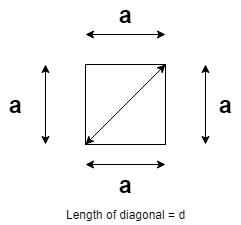Open in App
Not now

# Program to calculate length of diagonal of a square

• Difficulty Level : Medium
• Last Updated : 29 Apr, 2021

Given a positive integer S, the task is to find the length of diagonal of a square having sides of length S.

Examples:

Input: S = 10
Output: 14.1421
Explanation: The length of the diagonal of a square whose sides are of length 10 is 14.1421

Input: S = 24
Output: 33.9411

Approach: The given problem can be solved based on the mathematical relation between the length of sides of a square and the length of diagonal of a square as illustrated below:As visible from the above image, the diagonal and the two sides of the square form a right-angled triangle. Therefore, by applying Pythagoras Theorem:
(hypotenuse)2 = (base)2 + (perpendicular)2, where D and S are length of the diagonal and the square.

Therefore,
=>=>=>Therefore, simply calculate the length of the diagonal using the above-derived relation.

Below is the implementation of the above approach:

## C++

 // C++ program for the above approach #include  using namespace std;   // Function to find the length of the // diagonal of a square of a given side double findDiagonal(double s) {     return sqrt(2) * s; }   // Driver Code int main() {     double S = 10;     cout << findDiagonal(S);       return 0; }

## Java

 // Java program for the above approach import java.util.*;   class GFG{   // Function to find the length of the // diagonal of a square of a given side static double findDiagonal(double s) {     return (double)Math.sqrt(2) * s; }   // Driver Code public static void main(String[] args) {     double S = 10;           System.out.print(findDiagonal(S)); } }   // This code is contributed by splevel62

## Python3

 # Python3 program for the above approach import math   # Function to find the length of the # diagonal of a square of a given side def findDiagonal(s):           return math.sqrt(2) * s   # Driver Code if __name__ == "__main__":       S = 10           print(findDiagonal(S))   # This code is contributed by chitranayal

## C#

 // C# program for the above approach using System; public class GFG {   // Function to find the length of the // diagonal of a square of a given side static double findDiagonal(double s) {     return (double)Math.Sqrt(2) * s; }   // Driver Code public static void Main(String[] args) {     double S = 10;           Console.Write(findDiagonal(S)); } }   // This code is contributed by 29AjayKumar

## Javascript

 

Output:

14.1421

Time Complexity: O(1)
Auxiliary Space: O(1)

My Personal Notes arrow_drop_up
Related Articles